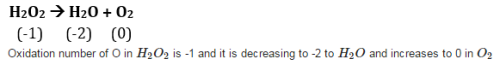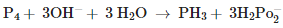Courses

# Test: Redox Reactions

## 25 Questions MCQ Test Chemistry for JEE | Test: Redox Reactions

Description
This mock test of Test: Redox Reactions for JEE helps you for every JEE entrance exam. This contains 25 Multiple Choice Questions for JEE Test: Redox Reactions (mcq) to study with solutions a complete question bank. The solved questions answers in this Test: Redox Reactions quiz give you a good mix of easy questions and tough questions. JEE students definitely take this Test: Redox Reactions exercise for a better result in the exam. You can find other Test: Redox Reactions extra questions, long questions & short questions for JEE on EduRev as well by searching above.
QUESTION: 1

### The electron releasing tendency of the metals, zinc, copper and silver is in the order:

Solution:

The electron releasing tendency of the metals, zinc, copper and silver is in the order Zn>Cu>Ag

QUESTION: 2

### In Daniell cell, direction of current is

Solution:

In Daniel cell direction of current is opposite to the direction of electron flow.

QUESTION: 3

### The oxidation number of hydrogen in LiH, NaH and CaH2 is

Solution:

alkaline earth metal have +2 oxidation state.

*Multiple options can be correct
QUESTION: 4

Which of the following electrodes will act as anodes, when connected to Standard Hydrogen Electrode?

Solution:

(a, b) Metals with negative standard reduction potential like Al and Fe will act as anode when connected to standard hydrogen electrode.

QUESTION: 5

A metal in a compound can be displaced by another metal in the uncombined state. Which metal is a better reducing agent in such a case?

Solution:

Reducing agent is one in which element undergo oxidation itself and make the other substance reduce.

QUESTION: 6

In the reaction of metallic cobalt placed in nickel sulphate solution, therein is a competition for release of electrons At equilibrium, chemical tests reveal that both Ni+2 (aq) and Co+2 (aq) are present at moderate concentrations. The result is that:

Solution:

Reaction will not go to completion. It will remain at equilibrium.

QUESTION: 7

The flow of current is possible in Daniel cell only

Solution:

The flow of current is possible only due to potential difference.

QUESTION: 8

Chlorine, bromine and iodine when combined with oxygen, have oxidation numbers

Solution:

If H is attached to metal then its oxidation state of H is -1.

QUESTION: 9

The decomposition of hydrogen peroxide to form water and oxygen is an example of

Solution:QUESTION: 10

Hydrogen is prepared from H2O by adding

Solution:

Ag and Au are less reactive than H( from reactivity series. So they can’t be considered). To form H2 from H2O, we need a reducing agent.

QUESTION: 11

Oxidation number denotes the oxidation state of an element in a compound ascertained on the basis that electron in a covalent bond belongs

Solution:

Oxidation number basically defines the charge present on element in combined form.

QUESTION: 12

In oxygen difluoride (OF2) and dioxygen difluoride(O2F2), the oxygen is assigned an oxidation number of

Solution:

Oxidation state of O in OF2 is +2 and O2F2 is +1.

QUESTION: 13

The oxidation number of an element in a compound is evaluated on the basis of certain rules. Which of the following rules is not correct in this respect?

Solution:

as oxygen is more electronegative than Cl,Br and I. So they have positive oxidation state.

QUESTION: 14

Identify the correct statements with reference to the given reactionSolution:

P4 is undergoing oxidation as well as reduction. As oxidation number of P4 is 0 in reactant and it increases to +3 in H2PO2− and decreases to -3 in PH3

QUESTION: 15

In the conversion of Br2 to Bro3- , the oxidation number of Br changes from

Solution:

In Br2 oxidation number of Br is 0 while in BrO3− Br has oxidation number of +5 as each O carries -2 charge and net charge is -1.

QUESTION: 16

The highest value of oxidation number changes from 1 to 7

Solution:

Across the 3rd period in the periodic table, oxidation n changes from K(19, +1) to Mn(25, +7).

QUESTION: 17

Oxidation number of 1/2 is assigned to oxygen atom in

Solution:

Superoxides have O2−

QUESTION: 18

The more positive the value of E0, the greater is the tendency of the species to get reduced. Using the standard electrode potential of redox couples given below find out which of the following is the strongest oxidising agent.

E0values : Fe3 + / Fe2+ = +0.77; I2(s)/l- = +0.54; cu2+/ Cu = +0.34; Ag+ / Ag = +0.80V

Solution:

Oxidation number of H is not always +1 . It can be -1 , 0.

QUESTION: 19

The electron releasing tendency of the metals, zinc, copper and silver is in the order:

Solution:

The electron releasing tendency of the metals, zinc, copper and silver is in the order Zn>Cu>Ag

QUESTION: 20

In the reaction
2H2O2 → 2H2O +O2

Solution:

This is a dispropotionation reaction.

QUESTION: 21

The oxidation number of oxygen in most compounds is

Solution:

Common oxidation state of oxygen is -2

QUESTION: 22

Assign oxidation number to P in NaH2PO4

Solution:

Oxidation state of P in NaH2PO4

QUESTION: 23

The oxidizing power of halogens increase in the order of

Solution:

I< Br< Cl< F

is the order of oxidising power.

QUESTION: 24

Identify the correct statement (s) in relation to the following reaction:  Zn+ 2HCl → ZnCl2 +H2

Solution:

Zn is oxidized to Zn+2 while H+ is reduced to H2. So H+ is acting as oxidant and Zn is acting as reductant.

QUESTION: 25

Consider the elements: Cs, Ne, I and F. Identify the element(s) that exhibits only negative oxidation state

Solution:

F has negative oxidation state as it is very electro negative.